# Confidence Intervals The Basics BPS chapter 14 2006

• Slides: 45Confidence Intervals: The Basics BPS chapter 14 © 2006 W. H. Freeman and CompanyStatistical Inference What is statistical inference on ? a) b) c) d) Drawing conclusions about a population mean based on information contained in a sample. Drawing conclusions about a sample mean based on information contained in a population. Drawing conclusions about a sample mean based on the measurements in that sample. Selecting a set of data from a large population.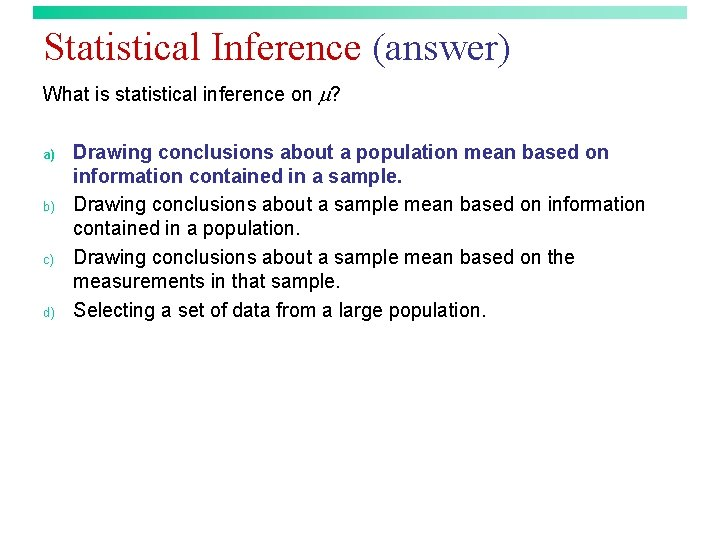Statistical Inference (answer) What is statistical inference on ? a) b) c) d) Drawing conclusions about a population mean based on information contained in a sample. Drawing conclusions about a sample mean based on information contained in a population. Drawing conclusions about a sample mean based on the measurements in that sample. Selecting a set of data from a large population.Inference The conditions for doing inference on using the standard normal distribution do NOT include: a) b) c) d) A simple random sample of size n. A normal population or sample size large enough to apply the Central Limit Theorem. A known value of .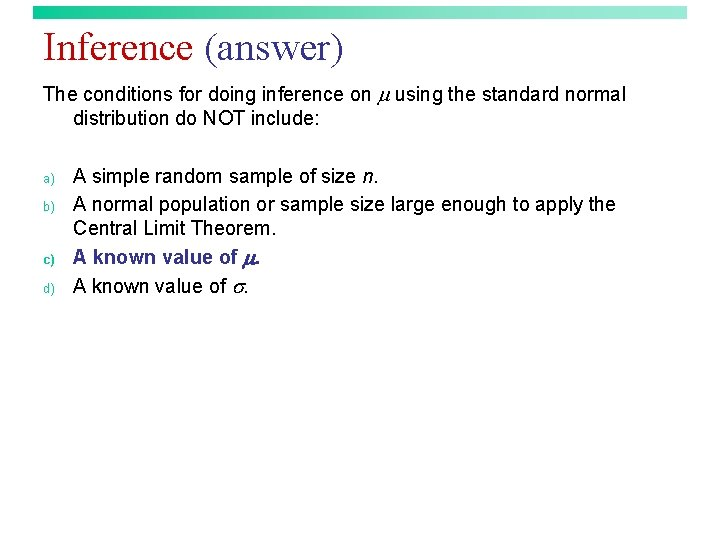Inference (answer) The conditions for doing inference on using the standard normal distribution do NOT include: a) b) c) d) A simple random sample of size n. A normal population or sample size large enough to apply the Central Limit Theorem. A known value of .Inference Why do we need a normal population or large sample size to do inference on ? a) b) c) d) So that the sampling distribution of is normal or approximately normal. So that the distribution of the sample data is normal or approximately normal. So that equals . So that is known.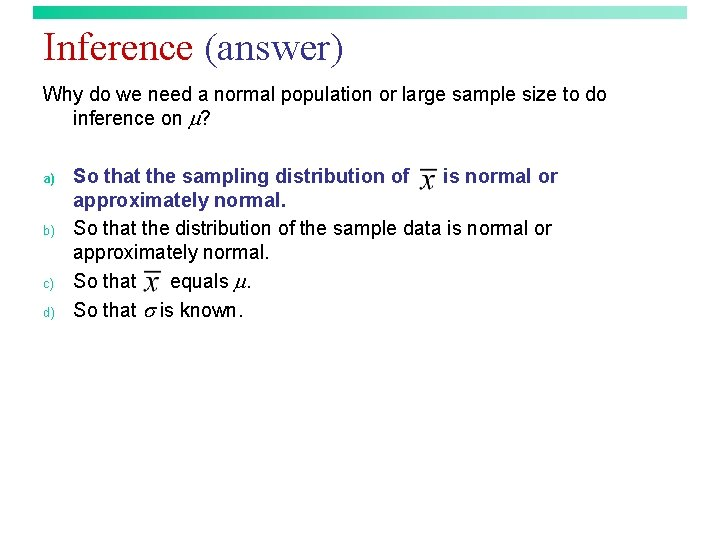Inference (answer) Why do we need a normal population or large sample size to do inference on ? a) b) c) d) So that the sampling distribution of is normal or approximately normal. So that the distribution of the sample data is normal or approximately normal. So that equals . So that is known.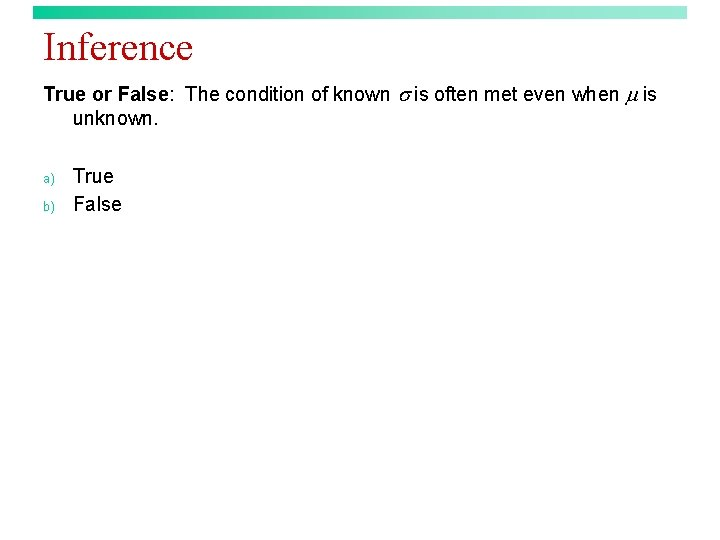Inference True or False: The condition of known is often met even when is unknown. a) b) True False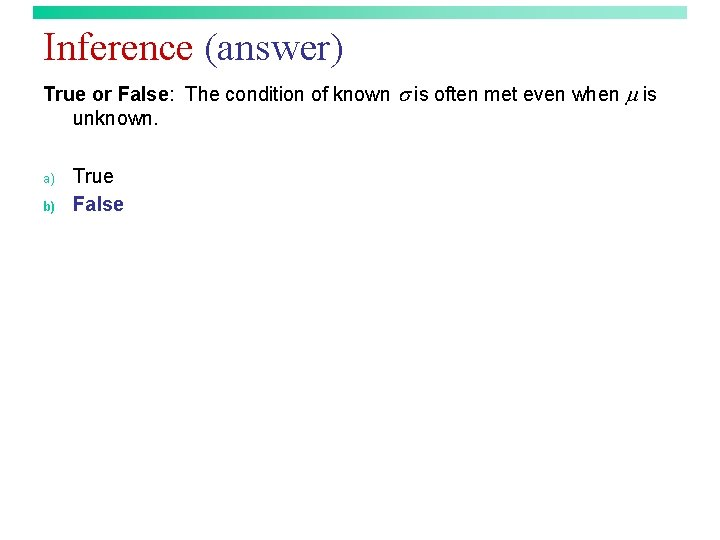Inference (answer) True or False: The condition of known is often met even when is unknown. a) b) True FalseConfidence Intervals The purpose of a confidence interval for is a) b) c) d) To give a range of reasonable values for the level of confidence. To give a range of reasonable values for the sample mean. To give a range of reasonable values for the population mean. To give a range of reasonable values for the difference between the sample mean and the population mean.Confidence Intervals (answer) The purpose of a confidence interval for is a) b) c) d) To give a range of reasonable values for the level of confidence. To give a range of reasonable values for the sample mean. To give a range of reasonable values for the population mean. To give a range of reasonable values for the difference between the sample mean and the population mean.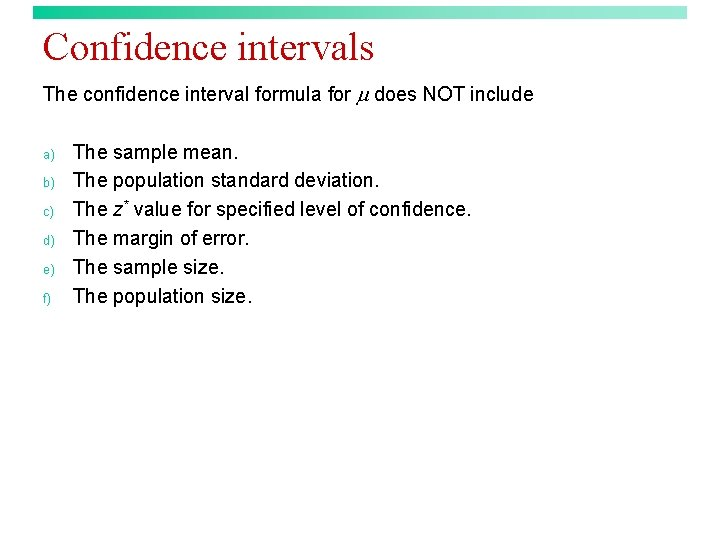Confidence intervals The confidence interval formula for does NOT include a) b) c) d) e) f) The sample mean. The population standard deviation. The z* value for specified level of confidence. The margin of error. The sample size. The population size.Confidence intervals (answer) The confidence interval formula for does NOT include a) b) c) d) e) f) The sample mean. The population standard deviation. The z* value for specified level of confidence. The margin of error. The sample size. The population size.Confidence intervals What do we hope to capture within a confidence interval? a) b) c) d) e) f) The unknown confidence level. The unknown parameter. The unknown statistic. The parameter estimate. The margin of error. The sample size.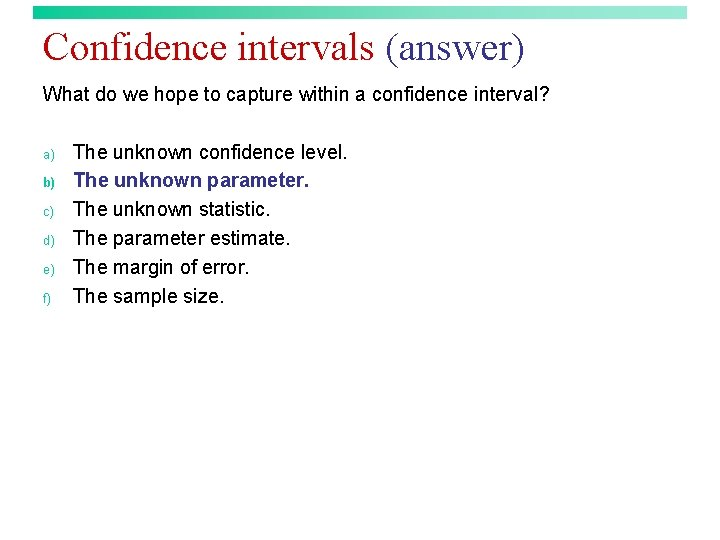Confidence intervals (answer) What do we hope to capture within a confidence interval? a) b) c) d) e) f) The unknown confidence level. The unknown parameter. The unknown statistic. The parameter estimate. The margin of error. The sample size.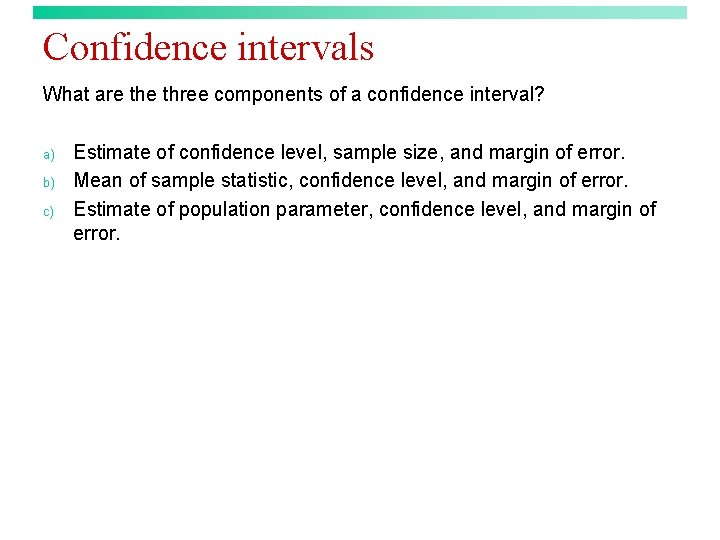Confidence intervals What are three components of a confidence interval? a) b) c) Estimate of confidence level, sample size, and margin of error. Mean of sample statistic, confidence level, and margin of error. Estimate of population parameter, confidence level, and margin of error.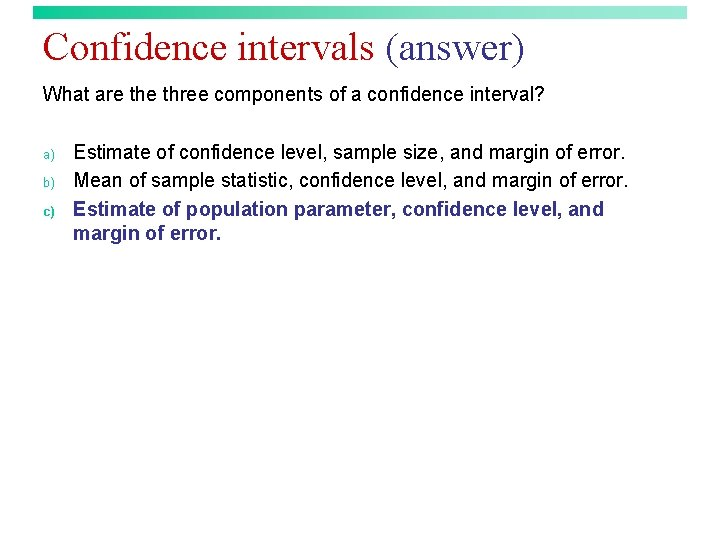Confidence intervals (answer) What are three components of a confidence interval? a) b) c) Estimate of confidence level, sample size, and margin of error. Mean of sample statistic, confidence level, and margin of error. Estimate of population parameter, confidence level, and margin of error.Confidence intervals Consider the following statement. “We are 90% confident that the population mean is between 344. 8 and 361. 2 pounds. ” Which of the following statements about this confidence interval interpretation is valid? a) b) c) d) This interpretation is incorrect because there is no statement of the true parameter in words. This interpretation is incorrect because the interval is not reported. This interpretation is incorrect because the confidence level is not reported. This is a correct reporting of the confidence interval.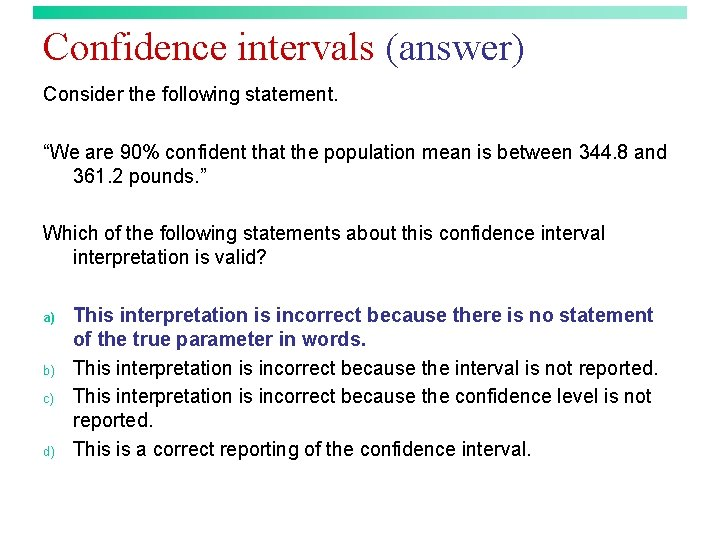Confidence intervals (answer) Consider the following statement. “We are 90% confident that the population mean is between 344. 8 and 361. 2 pounds. ” Which of the following statements about this confidence interval interpretation is valid? a) b) c) d) This interpretation is incorrect because there is no statement of the true parameter in words. This interpretation is incorrect because the interval is not reported. This interpretation is incorrect because the confidence level is not reported. This is a correct reporting of the confidence interval.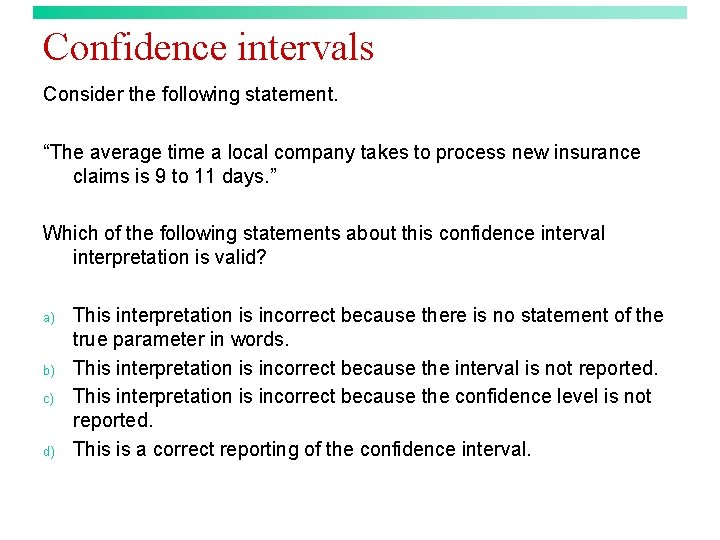Confidence intervals Consider the following statement. “The average time a local company takes to process new insurance claims is 9 to 11 days. ” Which of the following statements about this confidence interval interpretation is valid? a) b) c) d) This interpretation is incorrect because there is no statement of the true parameter in words. This interpretation is incorrect because the interval is not reported. This interpretation is incorrect because the confidence level is not reported. This is a correct reporting of the confidence interval.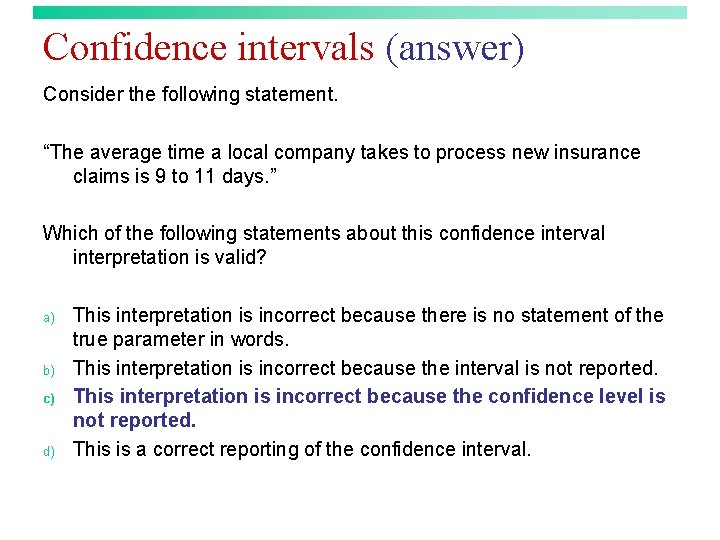Confidence intervals (answer) Consider the following statement. “The average time a local company takes to process new insurance claims is 9 to 11 days. ” Which of the following statements about this confidence interval interpretation is valid? a) b) c) d) This interpretation is incorrect because there is no statement of the true parameter in words. This interpretation is incorrect because the interval is not reported. This interpretation is incorrect because the confidence level is not reported. This is a correct reporting of the confidence interval.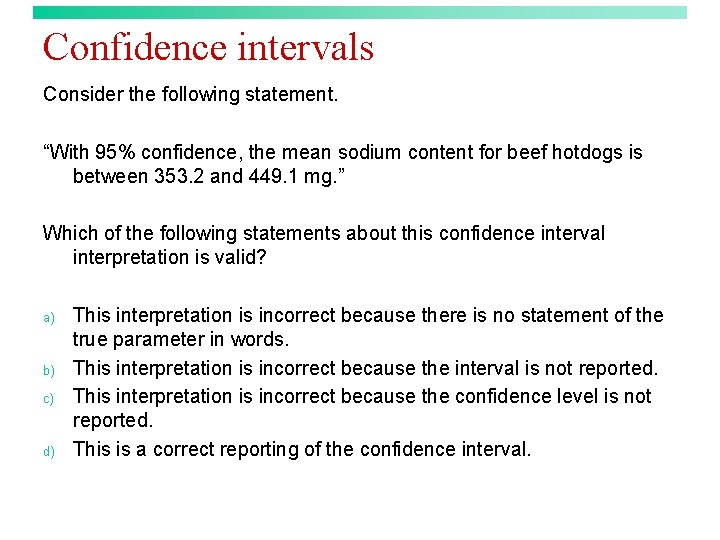Confidence intervals Consider the following statement. “With 95% confidence, the mean sodium content for beef hotdogs is between 353. 2 and 449. 1 mg. ” Which of the following statements about this confidence interval interpretation is valid? a) b) c) d) This interpretation is incorrect because there is no statement of the true parameter in words. This interpretation is incorrect because the interval is not reported. This interpretation is incorrect because the confidence level is not reported. This is a correct reporting of the confidence interval.Confidence intervals (answer) Consider the following statement. “With 95% confidence, the mean sodium content for beef hotdogs is between 353. 2 and 449. 1 mg. ” Which of the following statements about this confidence interval interpretation is valid? a) b) c) d) This interpretation is incorrect because there is no statement of the true parameter in words. This interpretation is incorrect because the interval is not reported. This interpretation is incorrect because the confidence level is not reported. This is a correct reporting of the confidence interval.Confidence intervals Consider the following statement. “With 99% confidence, the average time it takes passengers of a local airline to claim their luggage is 25 minutes. ” Which of the following statements about this confidence interval interpretation is valid? a) b) c) d) This interpretation is incorrect because there is no statement of the true parameter in words. This interpretation is incorrect because the interval is not reported. This interpretation is incorrect because the confidence level is not reported. This is a correct reporting of the confidence interval.Confidence intervals (answer) Consider the following statement. “With 99% confidence, the average time it takes passengers of a local airline to claim their luggage is 25 minutes. ” Which of the following statements about this confidence interval interpretation is valid? a) b) c) d) This interpretation is incorrect because there is no statement of the true parameter in words. This interpretation is incorrect because the interval is not reported. This interpretation is incorrect because the confidence level is not reported. This is a correct reporting of the confidence interval.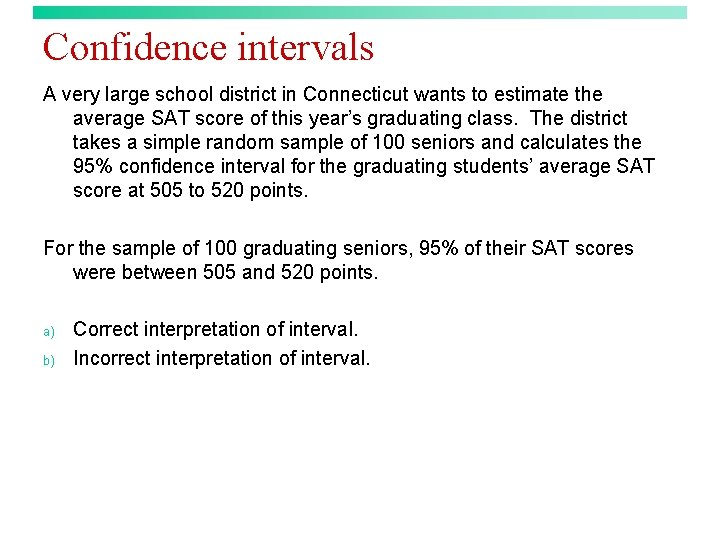Confidence intervals A very large school district in Connecticut wants to estimate the average SAT score of this year’s graduating class. The district takes a simple random sample of 100 seniors and calculates the 95% confidence interval for the graduating students’ average SAT score at 505 to 520 points. For the sample of 100 graduating seniors, 95% of their SAT scores were between 505 and 520 points. a) b) Correct interpretation of interval. Incorrect interpretation of interval.Confidence intervals (answer) A very large school district in Connecticut wants to estimate the average SAT score of this year’s graduating class. The district takes a simple random sample of 100 seniors and calculates the 95% confidence interval for the graduating students’ average SAT score at 505 to 520 points. For the sample of 100 graduating seniors, 95% of their SAT scores were between 505 and 520 points. a) b) Correct interpretation of interval. Incorrect interpretation of interval.Confidence intervals A very large school district in Connecticut wants to estimate the average SAT score of this year’s graduating class. The district takes a simple random sample of 100 seniors and calculates the 95% confidence interval for the graduating students’ average SAT score at 505 to 520 points. The school district can be 95% confident that the mean SAT score of the 100 students is contained in the interval of 505 to 520 points. a) b) Correct interpretation of interval. Incorrect interpretation of interval.Confidence intervals (answer) A very large school district in Connecticut wants to estimate the average SAT score of this year’s graduating class. The district takes a simple random sample of 100 seniors and calculates the 95% confidence interval for the graduating students’ average SAT score at 505 to 520 points. The school district can be 95% confident that the mean SAT score of the 100 students is contained in the interval of 505 to 520 points. a) b) Correct interpretation of interval. Incorrect interpretation of interval.Confidence intervals A very large school district in Connecticut wants to estimate the average SAT score of this year’s graduating class. The district takes a simple random sample of 100 seniors and calculates the 95% confidence interval for the graduating students’ average SAT score at 505 to 520 points. The interval of 505 to 520 points gives a range of reasonable values for the true average SAT score of the graduating students in the large Connecticut school district. The school district can be 95% confident this interval captures their graduating students’ average SAT score. a) b) Correct interpretation of interval. Incorrect interpretation of interval.Confidence intervals (answer) A very large school district in Connecticut wants to estimate the average SAT score of this year’s graduating class. The district takes a simple random sample of 100 seniors and calculates the 95% confidence interval for the graduating students’ average SAT score at 505 to 520 points. The interval of 505 to 520 points gives a range of reasonable values for the true average SAT score of the graduating students in the large Connecticut school district. The school district can be 95% confident this interval captures their graduating students’ average SAT score. a) b) Correct interpretation of interval. Incorrect interpretation of interval.Confidence intervals A very large school district in Connecticut wants to estimate the average SAT score of this year’s graduating class. The district takes a simple random sample of 100 seniors and calculates the 95% confidence interval for the graduating students’ average SAT score at 505 to 520 points. The probability that the interval of 505 to 520 points captures the large Connecticut school district’s graduating seniors’ average SAT score is 0. 95. a) b) Correct interpretation of interval. Incorrect interpretation of interval.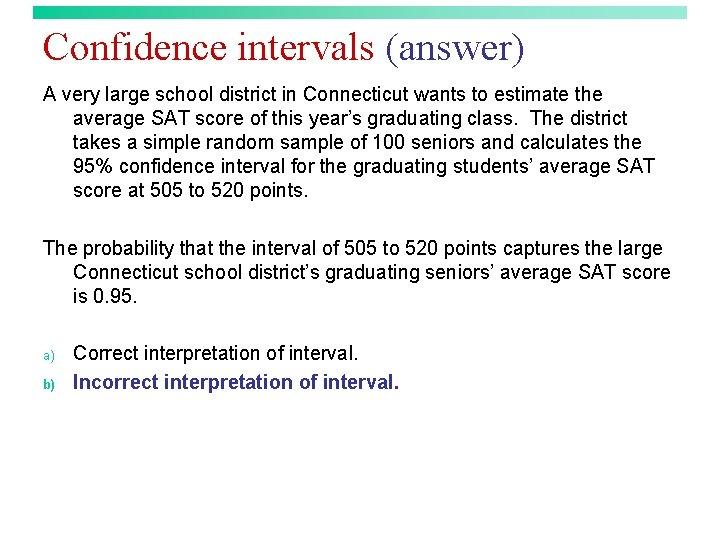Confidence intervals (answer) A very large school district in Connecticut wants to estimate the average SAT score of this year’s graduating class. The district takes a simple random sample of 100 seniors and calculates the 95% confidence interval for the graduating students’ average SAT score at 505 to 520 points. The probability that the interval of 505 to 520 points captures the large Connecticut school district’s graduating seniors’ average SAT score is 0. 95. a) b) Correct interpretation of interval. Incorrect interpretation of interval.Confidence intervals The probability that a 90% confidence interval for a population mean captures is a) b) c) d) 0. 0. 90. 1. Either 0 or 1—we do not know which.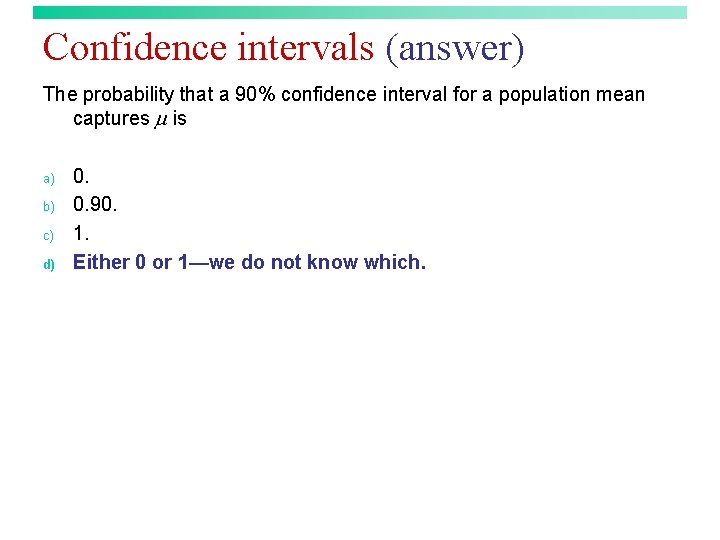Confidence intervals (answer) The probability that a 90% confidence interval for a population mean captures is a) b) c) d) 0. 0. 90. 1. Either 0 or 1—we do not know which.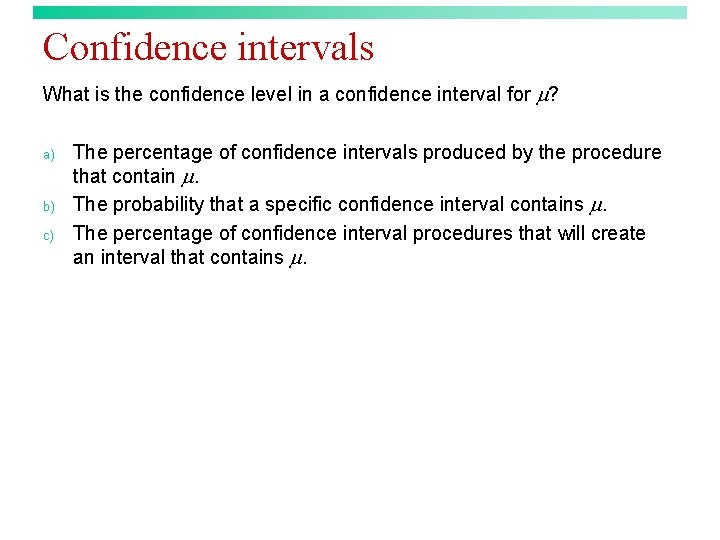Confidence intervals What is the confidence level in a confidence interval for ? a) b) c) The percentage of confidence intervals produced by the procedure that contain . The probability that a specific confidence interval contains . The percentage of confidence interval procedures that will create an interval that contains .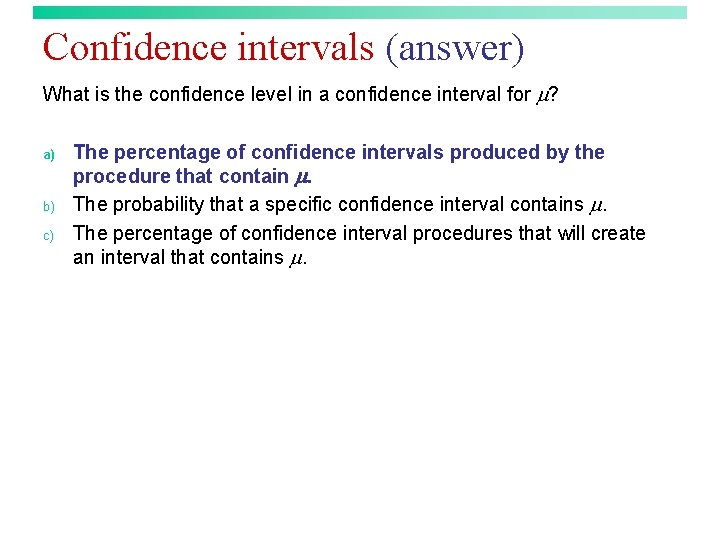Confidence intervals (answer) What is the confidence level in a confidence interval for ? a) b) c) The percentage of confidence intervals produced by the procedure that contain . The probability that a specific confidence interval contains . The percentage of confidence interval procedures that will create an interval that contains .Margin of error Increasing the confidence level will a) b) Increase the margin of error. Decrease the margin of error.Margin of error (answer) Increasing the confidence level will a) b) Increase the margin of error. Decrease the margin of error.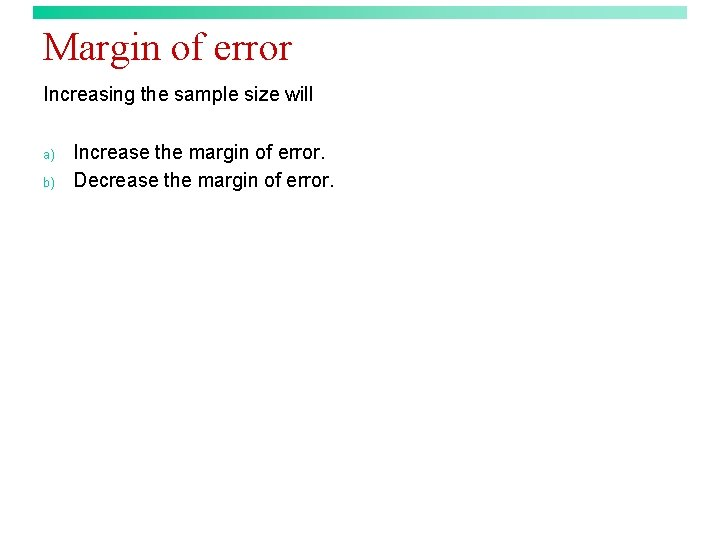Margin of error Increasing the sample size will a) b) Increase the margin of error. Decrease the margin of error.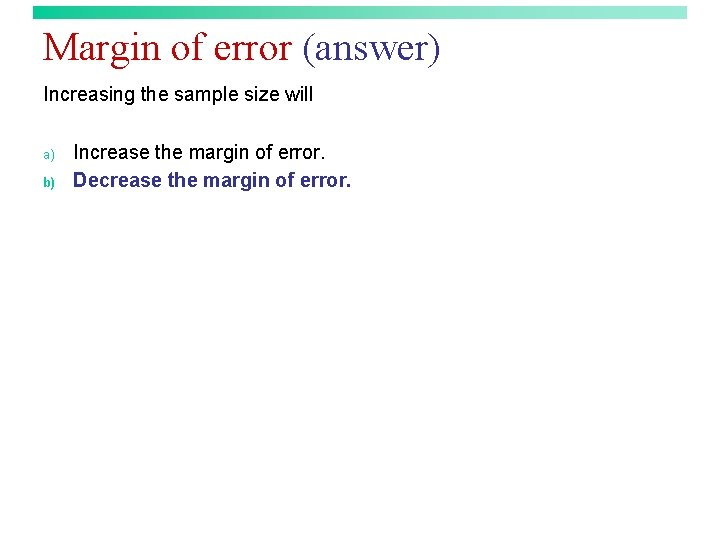Margin of error (answer) Increasing the sample size will a) b) Increase the margin of error. Decrease the margin of error.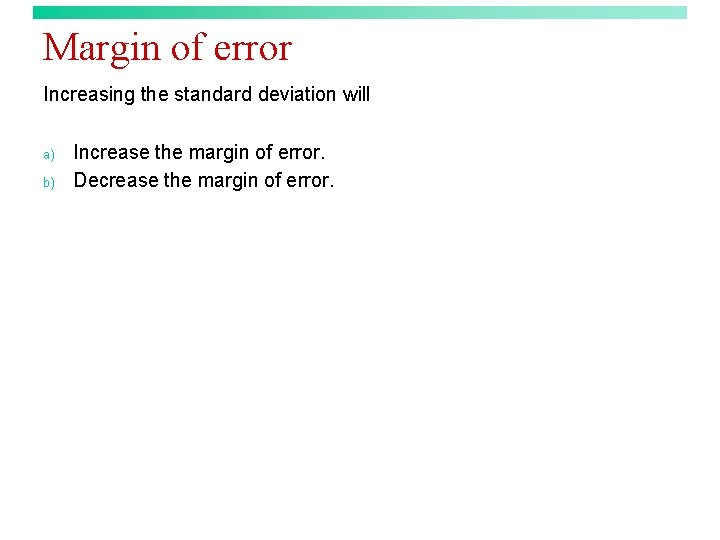Margin of error Increasing the standard deviation will a) b) Increase the margin of error. Decrease the margin of error.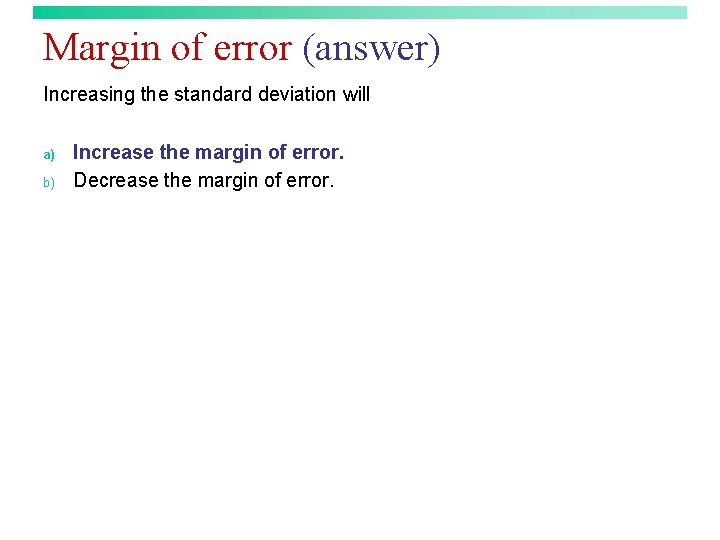Margin of error (answer) Increasing the standard deviation will a) b) Increase the margin of error. Decrease the margin of error.Margin of error Which of the following components of the margin of error in a confidence interval for does a researcher NOT have the chance to select? a) b) c) Confidence level. Sample size. Population standard deviation.Margin of error (answer) Which of the following components of the margin of error in a confidence interval for does a researcher NOT have the chance to select? a) b) c) Confidence level. Sample size. Population standard deviation.